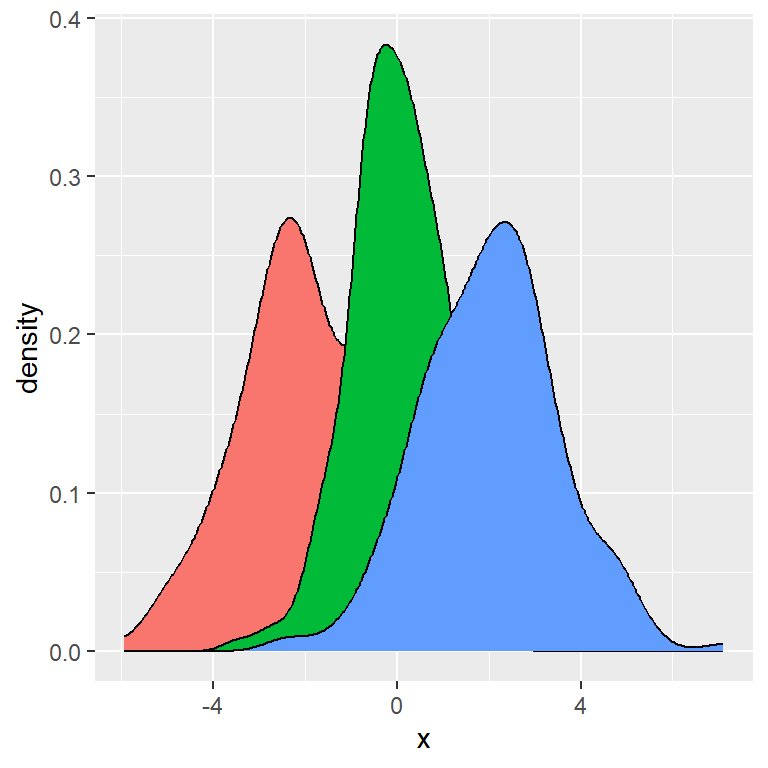# Density plot by group in ggplot2

## Sample data

The following data frame contains three normal distributions with different mean and standard deviation separated by group. This data will be used in all the examples of this tutorial.

``````# Data
set.seed(5)
x <- c(rnorm(200, mean = -2, 1.5),
rnorm(200, mean = 0, sd = 1),
rnorm(200, mean = 2, 1.5))
group <- c(rep("A", 200), rep("B", 200), rep("C", 200))
df <- data.frame(x, group)``````

## Density plot by group with `geom_density`

In order to create a density plot by group in ggplot you need to input the numerical variable and specify the grouping variable in `color` (or `colour`) argument inside `aes` and use `geom_density` function.

``````# install.packages("ggplot2")
library(ggplot2)

# Basic density plot in ggplot2
ggplot(df, aes(x = x, colour = group)) +
geom_density()``````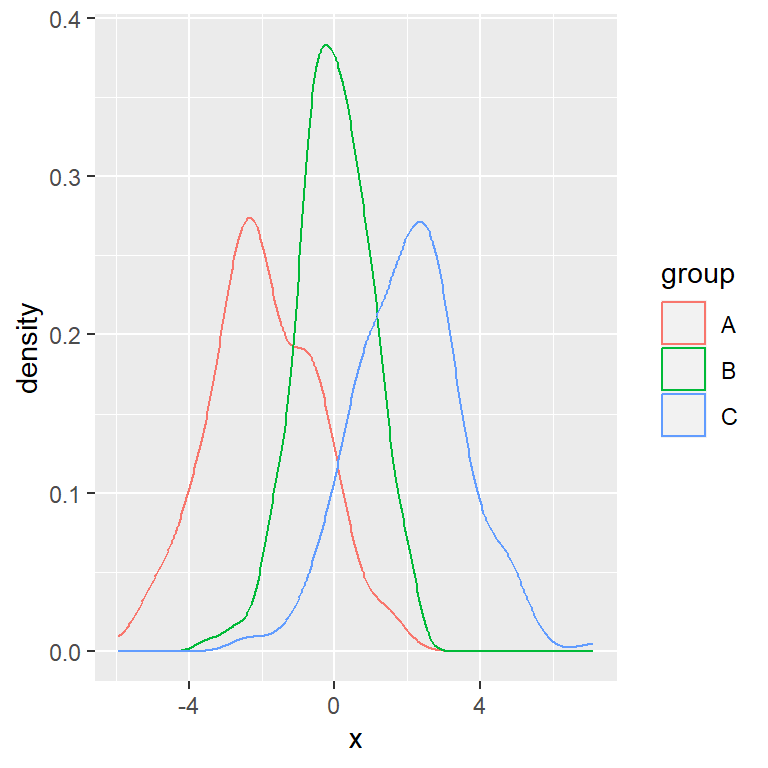The default color palette for the lines can be customized with `scale_color_manual` (or `scale_color_brewer`, for instance). You can also change the width and line type of the curves with `lwd` and `linetype`, respectively.

``````# install.packages("ggplot2")
library(ggplot2)

cols <- c("#F76D5E", "#FFFFBF", "#72D8FF")

# Basic density plot in ggplot2
ggplot(df, aes(x = x, colour = group)) +
geom_density(lwd = 1.2, linetype = 1) +
scale_color_manual(values = cols)``````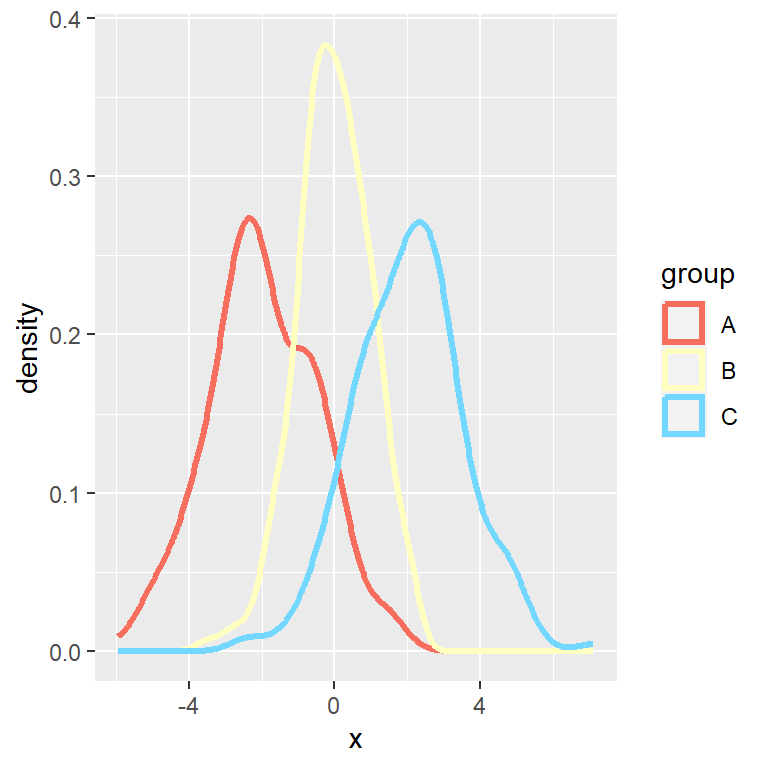### Fill the density areas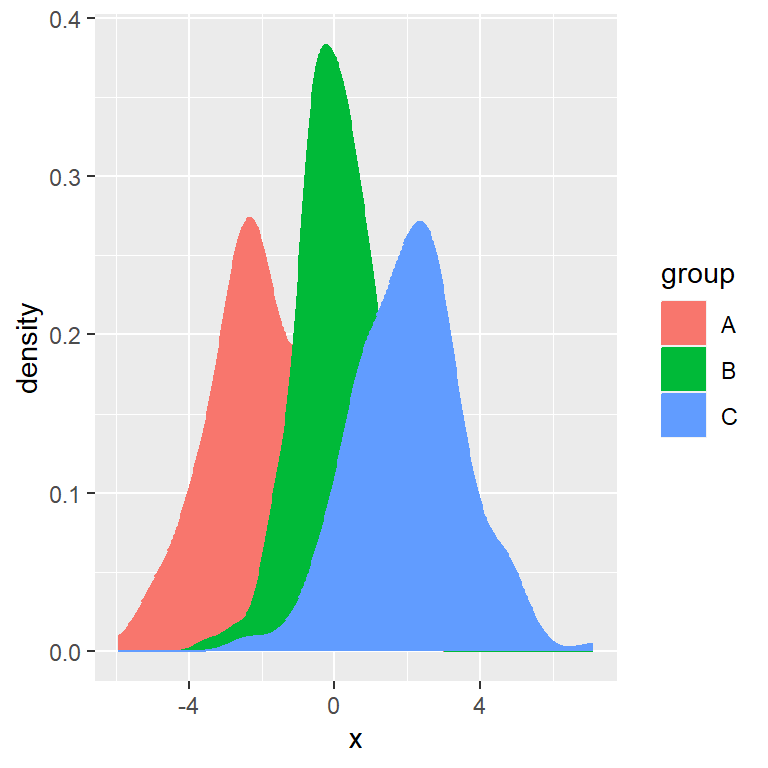If you also set the categorical variable to `fill` inside `aes` the areas under the curves will be filled with a color. Note that you can remove `colour = group` or set a custom color if you don’t want to color the lines by group.

``````# install.packages("ggplot2")
library(ggplot2)

# Basic density plot in ggplot2
ggplot(df, aes(x = x, colour = group, fill = group)) +
geom_density()``````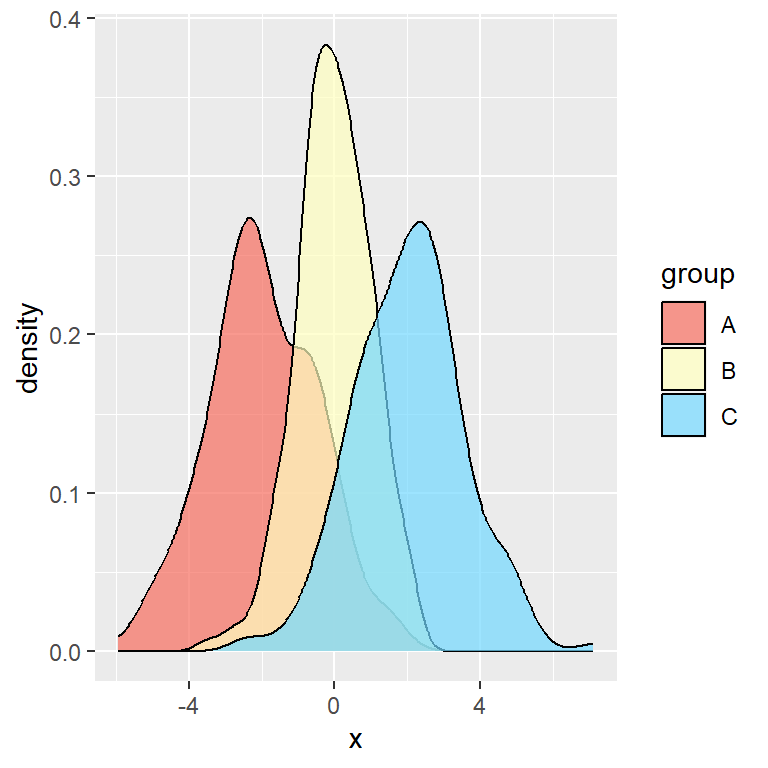Transparency and custom colors

You can modify the transparency of the areas with the `alpha` argument of `geom_density` and set custom colors with `scale_fill_manual`.

``````# install.packages("ggplot2")
library(ggplot2)

cols <- c("#F76D5E", "#FFFFBF", "#72D8FF")

# Basic density plot in ggplot2
ggplot(df, aes(x = x, fill = group)) +
geom_density(alpha = 0.7) +
scale_fill_manual(values = cols)``````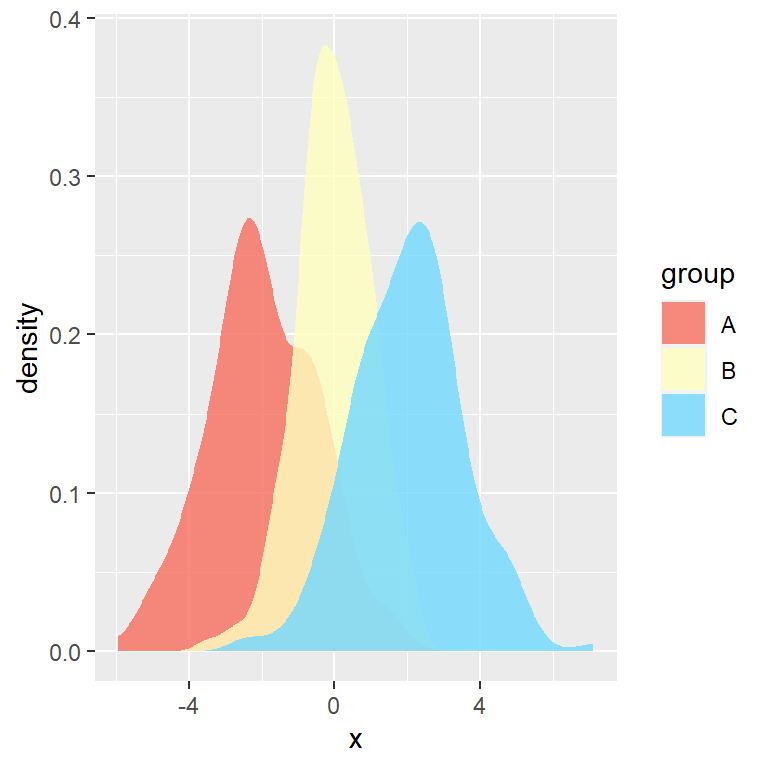Remove the lines

If you want to get rid of the lines and only show the area you can set `color = NA` inside `geom_density`.

``````# install.packages("ggplot2")
library(ggplot2)

cols <- c("#F76D5E", "#FFFFBF", "#72D8FF")

# Density areas without lines
ggplot(df, aes(x = x, fill = group)) +
geom_density(alpha = 0.8, color = NA) +
scale_fill_manual(values = cols)``````

### Legend customization

Custom title

The default title (the name of the categorical variable) can be customized with the following code.

``````# install.packages("ggplot2")
library(ggplot2)

# Basic density plot in ggplot2
ggplot(df, aes(x = x, fill = group)) +
geom_density() +
guides(fill = guide_legend(title = "Title"))``````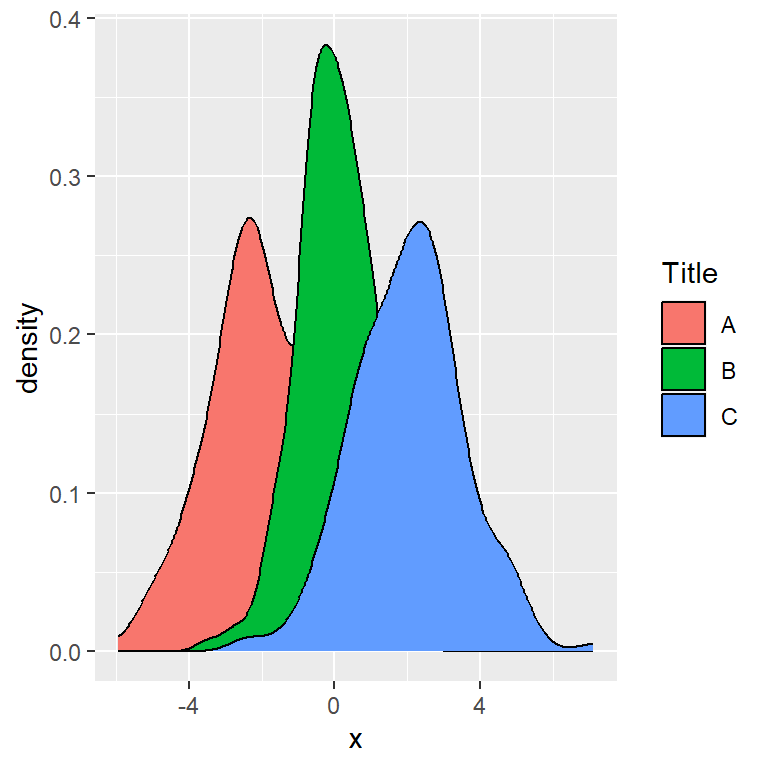Custom labels

You can also set custom key labels if you don’t want to use the name of your categories. Note that you can use `scale_fill_hue` if you only want to change the labels, but use the `labels` argument of `scale_fill_manual` if you also need to change the fill colors.

``````# install.packages("ggplot2")
library(ggplot2)

# Custom legend labels
ggplot(df, aes(x = x, fill = group)) +
geom_density() +
scale_fill_hue(labels = c("G1", "G2", "G3"))``````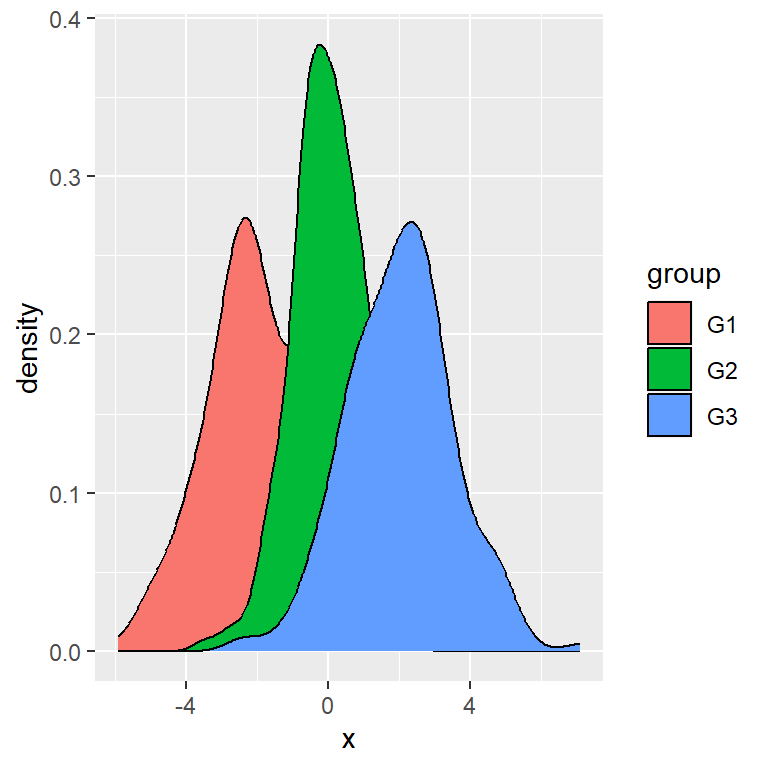Remove the legend

If you want to get rid of the legend, which appears by default, you can set its position to `"none"`.

``````# install.packages("ggplot2")
library(ggplot2)

# Basic density plot in ggplot2
ggplot(df, aes(x = x, fill = group)) +
geom_density() +
theme(legend.position = "none")``````